Subsections

# Free Energy Minima and Phases

## Phase Coexistence

Homogeneous state:
All part of my system are alike
Inhomogeneous state:
Some parts are different:Definition:
Macroscopic states, coexisting at different conditions, are called phases. Each phase exists in the thermodynamic limit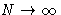Question:
Is microphase separation in co-polymers a true phase equilibrium?No, we do not have separate independent macroscopic phases! This is just one microscopically inhomogeneous macroscopic phase.

## Free Energy Minimum

### Problem

Suppose our system is at constant temperature. Total free energy is

 A(N1,N2,V1,V2,T) = A1(N1,V1,T) + A2(N2,V2,T) (1)

with(2)
We want to minimize (1) under conditions (2).

### Method of Lagrange Multipliers

We want to minimize f(x,y) under conditions g(x,y)=0. Construct a new function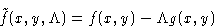and minimize this as function of independent variables x, y,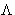. Minimizing bywe obtain g(x,y)=0, i.e.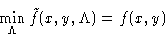Example:
I want to fence a rectangular lot of the given area A. How can I save money on the fence?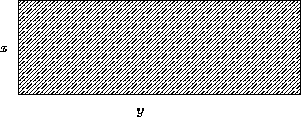Solution:
For an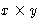lot the area is A=xy, the fence is f=2x+2y long. We want to minimize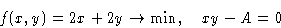We minimize: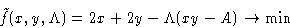and obtain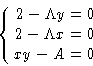The result is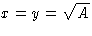--I'd better make a square lot.

### Minimization

To minimize free energy (1) under conditions (2), we minimize a new function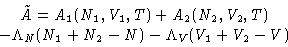(3)

#### Minimization with respect to V1 and V2: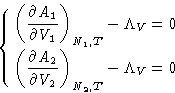The derivatives here are just (minus) pressures! We obtained:
1.
In equilibrium both phases have equal pressures (mechanic equilibrium) P1=P2=P
2.
Lagrange multiplier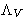is minus pressure of the system: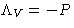#### Minimization with respect to N1 and N2:

1.
Both phases have equal chemical potentials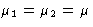2.
Lagrange multiplier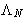is the chemical potential of the system:#### Conclusion

In coexisting phase P, T,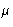are equal (as well as other thermodynamic fields).

#### Slightly Different View:

Let's forget about phase 2. The condition for N1 for phase 1 can be obtained from minimization a function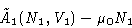with external pressure and chemical potential P0 and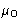.In equilibrium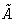is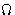-potential (we already derived this in previous lectures ).

#### Stability Condition:

The extremum should be a minimum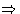second derivatives are positive!

### General Situation

Suppose we have an extensive variable xi. It has conjugated field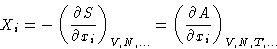In equilibrium Xi is constant in all phases, and the matrix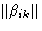with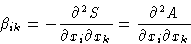is positive (in equilibrium S has maximum, and A minimum!). We can obtain this by minimizing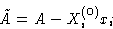with external value Xi(0)Next: Geometric Interpretation and Phase Up: Phase Equilibria Previous: Phase Equilibria

© 1997 Boris Veytsman and Michael Kotelyanskii
Thu Oct 2 21:02:12 EDT 1997